# Chapter 19: Volume and Surface Areas of Solids

## RS Aggarwal Class 10 Solutions Chapter 19

Question 1: Two cubes each of volume 27 cm3 are joined end to end to form a solid. Find the surface area of the resulting cuboid.

Sol:

As, volume of a cube = 27 cm3

(edge)3 = 27

Edge = $^{3}\sqrt{27}$

Edge = 3 cm

The length of the resulting cuboid, l = 3 + 3 = 6 cm,

Its breadth, b = 3 cm and its height, h = 3 cm.

Now, the surface area of the resulting cuboid = 2(lb + bh + hl)

= 2(6 x 3 + 3 x 3 + 3 x 6)

= 2(18+9+18) = 2 x 45 = 90 cm2

Question 2: The volume of a hemisphere is 2425 cm3. Find its curved surface area.

As, volume of hemisphere = 2425 $\frac{1}{2}cm^{3}$

$\Rightarrow \frac{2}{3}\prod r^{3}$ =2425 $\frac{1}{2}$

$\Rightarrow \frac{2}{3}\ X \frac{22}{7} X r^{3} = \frac{4851}{2}$

$\Rightarrow r^{3} = \frac{4851 \times 3 \times 7}{2\times 2\times 22}$

$\Rightarrow r^{3} = \frac{441 \times 3 \times 7}{2\times 2\times 2}$

$\Rightarrow r^{3} = \frac{21^{3}}{2^{3}}$

$\Rightarrow r = \frac{21}{2} cm$

So, the curved surface area of the hemisphere = $2\prod r^{2}$

$= 2\times \frac{22}{7}\times \frac{21}{2}\times \frac{21}{2}$ = 693 cm2

Question 3: If total surface area of a solid hemisphere is 462 cm2, find its volume.

As, the total surface area of the solid hemisphere = 462 cm2

$\Rightarrow 3\prod r^{2}= 462$

$\Rightarrow 3\times \frac{22}{7}\times r^{2}=462$

$\Rightarrow r^{2}=\frac{462\times 7}{3\times 22}$

$\Rightarrow r^{2}=49$

$\Rightarrow r^{2}=\sqrt{49}$

$\Rightarrow r= 7 cm$

Now, the volume of the solid hemisphere = $= \frac{2}{3}\prod r^{3}$

$= \frac{2}{3}\times \frac{22}{7}7\times 7\times 7$

$= \frac{2156}{3}cm^{3}$

$= 718\frac{2}{3}cm^{3}$ = 718.67 cm3

Question 4: A 5m wide cloth is used to make a conical tent of base diameter 14 m and height 24m. Find the cost used at the rate of Rs.25 per metre.

We have, the height of the cone, h = 24 m, the base diameter of the cone, d = 14m.

Also, the base rtadius of the cone, r = $= \frac{d}{2}=\frac{14}{2}=7 m$

The slant height of the cone, $l = \sqrt{h^{2}+r^{2}}$

$= \sqrt{24^{2}+7^{2}}$

$= \sqrt{576+49}$

$= \sqrt{625}$ = 25 m

The curved surface area of the tent = πrl

$= \frac{22}{7}\times 7\times 25$ = 550 m 2

$\Rightarrow$ the area of cloth required to make the tent = 550m2

$\Rightarrow$the length of the cloth = $\frac{550}{5}=110 m$

So, the cost of cloth used = 110 $\times 25= 2750$

Question 5: If the volumes of two cones are in the ratio of 1:4 and their diameter are in the ratio of 4:5, find the ratio of their heights.

Let r and R be the base radii, h and H be the heights, v and V be the volumes of the two given cones.

We have,

$\frac{2r}{2R}=\frac{4}{5}or\frac{r}{R}=\frac{4}{5}$ …(i)

And

$\frac{v}{V}=\frac{1}{4}$

$\Rightarrow \frac{(\frac{1}{3}\prod r^{2}h)}{(\frac{1}{3}\prod R^{2}H)}=\frac{1}{4}$

$\Rightarrow \frac{r^{2}h}{R^{2}H}=\frac{1}{4}$

$\Rightarrow \left ( \frac{r}{R} \right )^{2}\times \frac{h}{H}=\frac{1}{4}$

$\Rightarrow \left ( \frac{4}{5} \right )^{2}\times \frac{h}{H}=\frac{1}{4} [using(i)]$

$\Rightarrow \left ( \frac{16}{25} \right )\times \frac{h}{H}=\frac{1}{4} [using(i)]$

$\Rightarrow \frac{h}{H}=\frac{1\times 25}{4\times 16}$

$\Rightarrow \frac{h}{H}=\frac{25}{64}$

$Therefore, h\vdots H=25 \vdots 64$

So, the ratio of their heights is 25:64

Q.6: The slant height of the conical mountain is 2.5 Km and the area of its base is 1.54 $km^{2}$ .Find the height of the mountain.

Sol:

Let r, h and l be the base radius, and the slant height of the conical mountain, respectively.

As, the area of the base = 1.54 km2

$\Rightarrow \pi r^{2} = 1.54$

$\Rightarrow \frac {22} {7} \times r^{2} = 1.54$

$\Rightarrow r^{2} = \frac {1.54 \times 7} {22}$

$\Rightarrow r^{2} = 0.49$

$\Rightarrow r = 0.7 km$

Now,

$h = \sqrt{l^{2} – r^{2}}$

$= \sqrt{2.5^{2} – 0.7^{2}}$

$= \sqrt{6.25 – 0.49}$

$= \sqrt{5.76}$ = 2.4 km

So, the height of the mountain is2.4 km.

Q.7: The sum of the radius of the base and the height of a solid cylinder is 37 m. If the total surface area of the cylinder is 1628 square metre, find its volume.

Sol:

Let r and h be the base radius and the height of the solid cylinder, respectively.

We have,

(r + h) = 37 m

As, the total surface area of the cylinder = 1628 m2

$\Rightarrow 2\pi r \left ( r + h \right ) = 1628$

$\Rightarrow 2 \times \frac {22} {7} \times r \times 37 = 1628$

$\Rightarrow r = \frac {1628 \times 7} {2\times 22 \times 37}$

$\Rightarrow r = 7 m$

So, h = (37-7) = 30 m

Now, the volume of the solid cylinder = πr2h

= 22/7 × 7 × 7 × 30 = 4620 m3

Q.8: The surface area of the sphere is 2464 $cm^{2}$ . If its base radius be doubled, what will be the surface area of the new sphere?

Sol:

Let the radii of the given sphere and the new sphere be r and R, respectively.

We have,

R = 2r and the surface area of the given sphere = 2464 cm2

i.e. 4πr2 = 2464 cm2

The surface area of the new sphere = 4πR2

= 4π (2r) 2

= 4π (4r2)

= 4(4πr2)

= 4 × 2464

= 9856 cm2

So, the surface area of the new sphere is 9856 cm2

Q.9: A military tent of height 8.25m is in the form of the right circular cylinder of base diameter 30m and the height 5.5 surmounted by a right circular cone of the same base radius. Find the length of canvas used in making the tent, if the breadth of the canvas is 1.5m.

Sol:

We have,

The radii of base of the cone and cylinder, r = 15 m,

The height of the cylinder, h = 5.5 m,

The height of the tent = 8.25 m,

Also the height of the cone, H = 8.25 – 5.54 = 2.75 m

The slant height of the cone, l = $\sqrt {r^ {2} + H^ {2}}$

= $\sqrt {15^ {2} + 2.75^ {2}}$

= $\sqrt {225^ {2} + 7.5625^ {2}}$

= $\sqrt {232.5625}$ = 15.25 m

The area of the canvas used in making the tent = CSA of cylinder + CSA of cone

= 2πrh + πrl

= πr (2h + l)

= 22/7 × 15(2 × 5.5 + 15.25)

= 22/7 × 15(11 + 15.25)

= 22/7 × 15 × 26.25

= 1237.5 m2

As the width of the canvas = 1.5 m

So, the length of the canvas = 237.5/1.5 = 825 m

Hence, the length of the tent used for making the tent is 825 m.

Q.10: A tent is in the shape of a right circular cylinder up to a height of 3 m and conical above it. The total height of the tent is 13.5 metre and the radius of its base is 14 metre. Find the cost of the cloth required to make the tent at the rate of Rs. 80 per square metre.

Sol 10: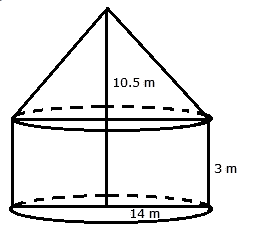Radius of the cylinder = 14 m

And its height = 3 m

Radius of cone = 14 m

And its height = 10.5 m

Let l be the slant height

l2 = (14)2 + (10.5)2

l2  = (196 + 110.25) m2

l2 = 306.25 m2

l = $\sqrt{306.25} m^{2}$

= 17.5 m

Curved surface area of tent

= (curved area of cylinder + curved surface area of cone)

= 2?rh + ?rl

= $[(2 \times \frac{22}{7} \times 14 \times 3) \, + \, (\frac{22}{7} \times 14 \times 17.5) ] m^{2}$

= (264 + 770)m2 = 1034 m2

Hence the curved surface area of the tent = 1034

Cost of canvas = Rs.(1034 * 80) = Rs. 82720

Q.11: A circus tent is cylindrical to a height of 3 m and conical above it. If its base radius is 52.5 m and the slant height of the conical portion is 53 m.Find the area of the canvas needed to make the tent.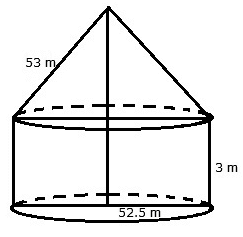For the cylindrical portion, we have radius = 52.5 m and height = 3 m

For the conical portion, we have radius = 52.5 m

And slant height = 53 m

Area of canvas = 2rh + rl = r(2h + l)

= $\frac{22}{7} \times 52.5 \times (2 \times 3 + 53) m^{2}$

= $22 \times \frac{15}{2} \times 59 m^{2} = 9735 m^{2}$

Q.12: A rocket is in the form of circular cylinder closed at the lower end and a cone of the same radius is attached to the top . The radius of the cylinder is 2.5 m, its height is 21 m and the slant height of the cone is 8 m. Calculate the total surface area of the rocket.

Sol: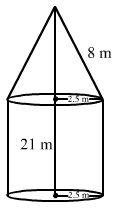Radius of cylinder = 2.5 m

Height of cylinder = 21 m

Slant height of cone = 8 m

Radius of cone = 2.5 m

Total surface area of the rocket = (curved surface area of cone + curved surface area of cylinder + area of base)

= (?rl + 2?rh + ?r2)

Where l = 8 m, h = 21 m, r = 2.5 m

= $\frac{22}{7} \times 2.5 \times 8 + 2 \times \frac{22}{7} \times 2.5 \times 21 + \frac {22}{7} \times 2.5 \times 2.5) m^{2}$

= (62.85 + 330 + 19.64) m2 = 412.5m2

Q.13: A solid is in the shape of a cone surmounted on a hemisphere the radius of each of them being 3.5 cm and the total height of the solid is 9.5 cm. Find the volume of the solid.

Sol: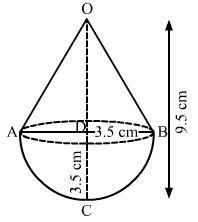We have,

Radius of the cone = Radius of the hemisphere = r = 3.5 cm or AD = BD = CD = 3.5 cm,

Total height of the solid, OC = 9.5 cm

= OD + CD = 9.5

= OD + 3.5 = 9.5

= OD = 6cm

= Height of cone, h = 6 cm

Now,

Volume of solid = volume of cone + volume of hemisphere

= $\frac {1} {3} \pi r^ {2} h + \frac {2} {3} \pi r^ {3}$

= $\frac {1} {3} \pi r^ {2} \left (h + 2r \right )$

= $\frac{1}{3} \times \frac{22}{7} \times 3.5 \times 3.5 \times \left ( 6 + 2 \times 3.5 \right )$

= $\frac {1} {3} \times \frac {22} {7} \times 3.5 \times 3.5 \times \left (6 + 7 \right )$

= $\frac {1} {3} \times \frac {22} {7} \times 3.5 \times 3.5 \times \left (13 \right )$

= $\frac {500.5} {3}$

= 166.83 cm3

So, the volume of the solid is 166.83 cm3

Q.14: A toy is in the form of a cone mounted on a hemisphere, of same radius 7 cm, if the total height of the toy is 31 cm. Find the total surface area.

Sol: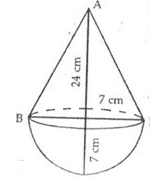Height of cone = h = 24 cm

Its radius = 7 cm

Hence, slant height = $\sqrt{(24)^{2} + 7^{2}}$ = $\sqrt{576 + 49}$ = $\sqrt{625} = 25 cm$

Total surface area of toy = (?rl + 2?r2)

= ?r(l + 2r) $\frac{22}{7} \times 7 \times (25 + 14)$ = 858 cm2

Q.15: A toy is in the shape of a cone mounted on a hemisphere of same base radius. If the volume of the toy is 231 $cm^ {3}$and its diameter is 7 cm, find the height of the toy.

Sol: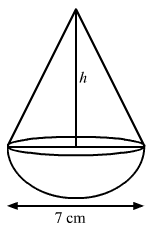We have,

Base radius of cone = base radius of hemisphere = r = 7/2 = 3.5 cm,

As, the volume of the toy = 231 cm3

= Volume of cone + volume of hemisphere = 231

$\Rightarrow \frac {1} {3} \pi r^ {2} h + \frac{2}{3}\pi r^ {3} = 231$

$\Rightarrow \frac {1} {3} \pi r^ {2} \left (h + 2r \right) = 231$

$\Rightarrow \frac {1} {3} \times \frac {22} {7} \times 3.5 \times 3.5 \times \left (h + 2 \times 3.5 \right) = 231$

$\Rightarrow \frac {38.5} {3} \times \left ( h + 7 \right ) = 231$

$\Rightarrow \left (h + 7 \right ) = \frac {231 \times 3} {38.5}$

$\Rightarrow \left ( h + 7 \right ) = 18$

$\Rightarrow h = 18 – 7$

$\Rightarrow h = 11 \; cm$

So, the height of the toy = h + r = 11 + 3.5 = 14.5 cm

Q.16: A cylindrical container of radius 6 cm and height 15 cm is filled with ice cream. The whole ice cream has to be distributed to 10 children in equal cones with hemispherical tops. If the height of the conical portion is 4 times the radius of its base. Find the radius and ice cream cone.

Sol: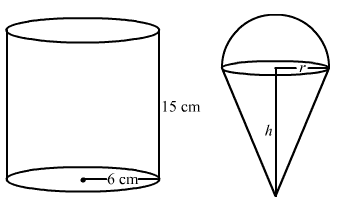We have,

The base radius of the cylindrical container, R = 6 cm,

The height of the container, H = 15 cm,

Let the base radius and the height of the ice-cream cone be r and h, respectively.

Also, h = 4r

Now, the volume of the cylindrical container = πR2H

= 22/7 × 6 × 6 × 15 = 11880/ 7 cm3

The volume of the ice cream – cream distributed to 10 children = 11880/7 cm3

= 10 × volume of ice cream cone = 11880/7

= 10 × (volume of cone + volume of hemisphere) = 11880/7

$\Rightarrow 10 \times \left (\frac {1} {3} \pi r^ {2} h + \frac {2} {3} \pi r^ {3} \right) = \frac {11880} {7}$

$\Rightarrow 10 \times \left ( \frac {1}{3} \pi r^ {2} \times 4r + \frac {2} {3} \pi r^{3} \right ) = \frac {11880} {7}$

$\Rightarrow 10 \times \left ( \frac {4} {3} \pi r^ {3} + \frac {2} {3} \pi r^ {3} \right ) = \frac {11880} {7}$

$\Rightarrow 10 \times \left ( \frac {6} {3} \pi r^ {3} \right ) = \frac {11880} {7}$

$\Rightarrow 10 \times 2 \times \frac {22} {7} \times r^ {3} = \frac {11880} {7}$

$\Rightarrow r^ {3} = \frac {11880 \times 7} {7\times 10 \times 2 \times 22}$

$\Rightarrow r^ {3} = 27$

$\Rightarrow r = \sqrt  {27}$

Therefore, r = 3 cm

So, the radius of the ice cream cone is 3 cm.

Q.17: A vessel is in the form of a hemispherical bowl mountain surmounted by a hollow cylinder. The diameter of the hemisphere is 21 cm and the total height of the vessel is 14.5 cm. Find its capacity.

Sol:

Radius of hemisphere = 10.5 cm

Height of cylinder = (14.5 – 10.5) cm = 4 cm

Radius of cylinder = 10.5 cm

Capacity = Volume of cylinder + Volume of hemisphere

= $(\pi r^ {2} h + \frac{2}{3} \pi r^{3} ) cm^{3} = \pi r^{2} (h + \frac{2}{3} r) cm^{3}$

= $\frac{22}{7} \times 10.5 \times 10.5 \times (4 + \frac{2}{3} \times 10.5) cm^ {3}$ = 3811.5 cm2

Q.18: A toy is in the form of a cylinder with hemispherical end. If the whole length of the toy is 90 cm and its diameter is 42 cm. Find the cost of painting the toy at the rate of 70 paisa per square centimetre.

Sol: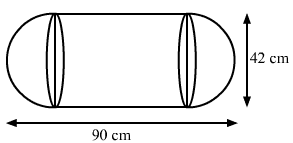We have,

The total height of the toy = 90 cm and the radius of the toy, r = 42/2 = 21 cm

Also, the height of the cylinder, h = 90 – 42 = 48 cm

Now, the total surface area of the toy = CSA of cylinder + 2 × CSA of a hemisphere

= 2πrh + 2 × 2πr2 = 2πr (h + 2r)

= 2 × 22/7 × 21 × (48 + 2 × 21)

= 44 × 3 × (48 + 42) = 44 × 3 × 90 = 11880 cm2

So, the cost of painting the toy = 11880 × 70/100 = Rs 8316

#### Practise This Question

3 people A, B and C are sitting in a circular fashion. Find the probability that A and B do not sit together.